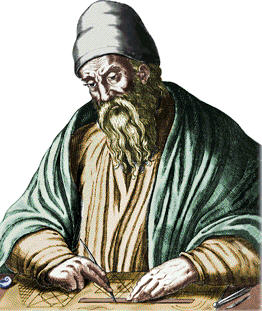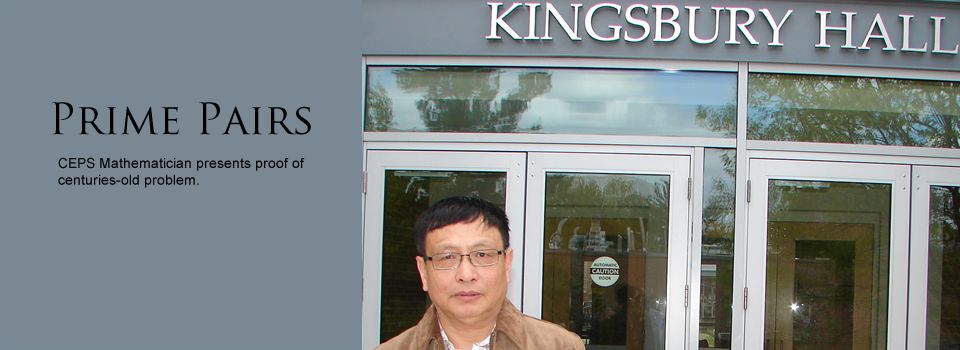# The Mystery of the Primes

Yesterday, I had the fortunate opportunity to spend some time with one of the most unusual mathematicians I have ever met--a middle-aged man born in China who, quietly, without talking much or making statements at international conferences, just solved one of the most important long-standing enigmas in mathematics: uncovering a key property of prime numbers.

A prime number is one that can be divided without a remainder only by 1 or by itself. The first prime is 2 (for technical reasons, 1 is not considered a prime), the next one is 3, and then come, in succession, 5, 7, 11, 13, 17, 19, and so on. We see, for example, that 19 is not divisible (without a remainder) by any integer other than 1 or 19; as compared with the composite (meaning non-prime) number 20, which is divisible by 2, 4, 5, and 10 (in addition to 1 and 20). Prime numbers are the building-blocks of our numbers, because every positive integer is either a prime number or a product of primes. The number 666 ("The number of the beast" of the Apocalypse) is composite and is the product of the primes 2, 3, again 3, and 37. The primes are thus the basic elements of our entire number system we use for everything. And, by themselves, they are not of academic interest only. To give you an idea about how important prime numbers are, every time you use your ATM card, the bank's computer verifies that you are who you claim you are through an algorithm that breaks down a huge number into a unique product of two known prime numbers (which is something that cannot quickly be done by trial and error, as an electronically-savvy thief might try to do).

The primes have been known since early antiquity and fascinated the ancient Greeks, who marveled at their nature. What is interesting about prime numbers is that they become rarer as we progress through the integers. The numbers of primes in the first five blocks of 1,000 integers are: 168, 135, 127, 120, and 119. And the block of 1,000 numbers just below and including 10 million has only 53 primes. So we see that the primes decrease in number. Do they ever end? Is there a point in the number system after which there are no longer any prime numbers, as we might expect from noticing the average decrease in the density of the primes?

The answer, given by the Greek mathematician Euclid of Alexandria, as far back as 300 B.C., is no. Euclid proved that there are, in fact, infinitely many prime numbers! The proof is so elegant and understandable by anyone who can do basic arithmetic that I feel compelled to present it here.Euclid of Alexandria (Wikimedia Commons)

So Euclid says: Let's assume that there are only a finite number of primes. In that case, there must be a last prime, after which the numbers, all the way to infinity, are composite (i.e., products of prime numbers, such as 666 above). Euclid then says: Let me call that last prime p. Now, so cleverly, Euclid writes down the following number: 2 x 3 x 5 x 7 x 11 x...x p + 1, that is, the product of all the prime numbers, from the first one, 2, to the last one, p, plus the number 1. And he asks the question: Is this new number a prime or not? If it is a prime, then we have just found a prime that is greater than the assumed largest prime, p--which is a contradiction! And if this new number is not a prime, then by the definition of a composite number it must be divisible by at least one of the prime numbers, i.e., by 2, by 3, by 5, by 7, by 11,...or by p. Call the prime number that, without remainder, divides our number above q. But when we actually do the division, we must get a remainder of 1/q, because we have that "1" added to the product of primes above. So in fact q does not divide our number without a remainder. Our number, therefore, cannot be composite. So here, too, we have a contradiction! This contradiction of the assumption that there exists a "last" prime number establishes the theorem: There are infinitely many prime numbers!

Now, primes like company. Often we see two primes that are "neighbors" because they are separated by only one number (the separation by one is necessary because, after 2, all primes must be odd, and after an odd number there must come an even number): 5 and 7, 11 and 13, 17 and 19, and so on. So mathematicians have asked the question: Are there only a finite set of such pairs of primes, or do pairs of primes continue to infinity? And, are there always primes separated by a length of numbers, or do primes with a given "gap" between them eventually disappear as the primes become rarer? A seminal textbook on number theory, An Introduction to the Theory of Numbers, by the prominent British mathematician G. H. Hardy (who brought to Cambridge the Indian genius Ramanujan, for those who know that story) and his coauthor E. M. Wright, says about such conjectures that (Oxford, 1998, p. 5): "their proof or disproof is at present beyond the resources of mathematics."

Beyond the resources of mathematics? Apparently, not everyone agreed to be defeated by numbers, even if this statement was made by one of the most famous mathematicians (now deceased).

I spent the academic year 2007-8 teaching mathematics at the University of New Hampshire. And there was something that irritated me. Often, while I was teaching my class, a person would pace outside my door, distracting my students. He was like a ghost, an apparition suddenly materializing in the corridor, staring into the room, apparently deep in thought, turning on his heels, pacing in the corridor, then coming back, again peering into the classroom and startling my students, and then disappearing from view. It went on for months. So I discreetly asked my department chair, Eric Grinberg, about it, and he said: "Oh, that's Tom--Yitang Zhang; he is working on a very important theorem in number theory. Haven't you met?" And I realized that I had indeed met him when I joined the department for a year, but that he was so quiet and untalkative that I didn't remember him. Now, UNH is an excellent school, but it only had one mathematician who was famous for proving a major theorem, Ken Appel (he has died recently), who in 1976 co-proved the famous four-color map theorem, so I didn't think that it was likely that someone else in this department would do something similar. And I learned to ignore the distractions and just teach my class.Tom Zhang of New Hampshire (Univ. of New Hampshire)

Then last week I got an e-mail from Eric, who'd since moved to the University of Massachusetts, Boston: "Come hear Tom Zhang present his theorem Tuesday at 2 p.m." The announcement he attached said that Zhang had recently stunned the world of mathematics by proving an immensely important property of prime numbers. Tom was virtually unknown to mathematicians and had not published in many years. His proof of a big theorem, recently accepted for publication by the leading math journal Annals of Mathematics has taken the math world by surprise. So I came, eager to see how he did it, accompanied by my friend Marina Ville, a mathematician visiting from the University of Tours, in France.

We weren't disappointed. Tom Zhang was no longer the apparition haunting my class. He was a flesh-and-blood mathematician, full of energy and eloquence, and he gave us a brilliant presentation of his proof of what is known as the "bounded gap conjecture." He showed that there are infinitely many consecutive prime numbers that differ by no more than 70 million. This means that the gaps between prime numbers do not necessarily increase indefinitely as the numbers grow--as we might suspect from the fact that the primes become rarer as the numbers progress. There are infinitely many prime number pairs whose separation is at most 70,000,000. This is a huge breakthrough in mathematics, and mathematicians hope that Zhang's methods should apply to further proving that there are infinitely many prime pairs separated by one intervening number only.

Immediately, one wonders: why 70 million? Where does such a number come from?--It seems so arbitrary. In fact, Zhang's technical proof employed a bound on key mathematical terms in his equation, a number raised to the power 1/4 + 1/1168. The power 1/4 (meaning the fourth root of a number) had been employed by earlier mathematicians working on this hard problem. Zhang's great breakthrough was a mathematical tightrope walk: in order to prove a result about consecutive primes, he had to raise the power 1/4 just slightly, without disturbing other terms. He finally managed to do this--satisfy the competing needs of different terms in his equation--by increasing the exponent 1/4 by the magical amount 1/1168. "Why this number?" someone in the audience asked him. "I was tired," he answered, "and this number worked." We all laughed. It was the use of the number 1/1168 in an exponent that led to the maximal gap of 70,000,000. Presumably, when he is not tired, Zhang may improve the bound, lowering the 70 million to smaller gaps between primes, and perhaps even solve the more specific "twin prime" conjecture on the infinitude of pairs of primes separated by one number.

Eric is a gracious host, and after Zhang's talk, he invited us for a tour of the UMB campus, situated on a tongue of land that juts into Massachusetts Bay and borders the John F. Kennedy Library. As we walked by the water's edge on a beautiful June day, a group of six mathematicians, we reached the Library and saw Kennedy's wooden sailboat displayed on the grass in front of it. Tom kept leaving our group to meditate alone for a few minutes at a time. "He's already working on his next theorem," Marina said to me. "Sure," I said, "and admiring JFK's pretty sailboat is certainly more inspiring than staring into a classroom."JFK's Sailboat